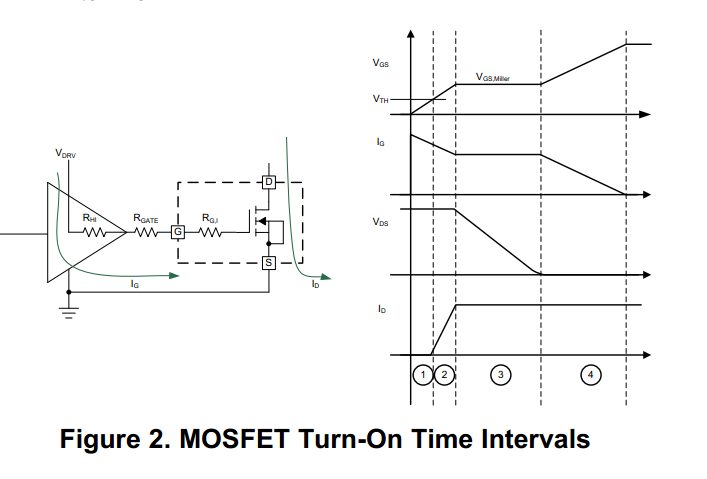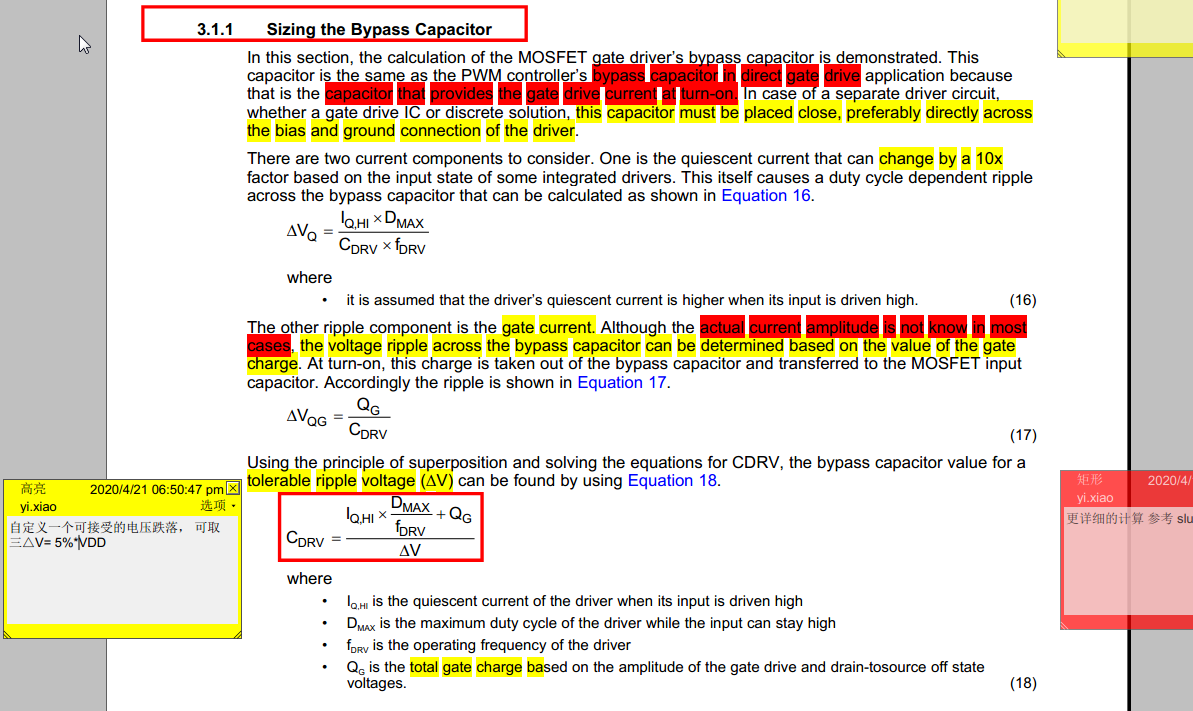If you have a related question, please click the "Ask a related question" button in the top right corner. The newly created question will be automatically linked to this question.

• Resolved

# UCC38C43: how to determine MOSFET gate drive peak current

Part Number: UCC38C43

Hi there,

I consider to use UCC38C43 in simple flyback design to drive Vishay mosfet SIR872A, and have some questions about how can we calculate the peak and average gate current of the mosfet.

Design Spec: Vdrv(3843) =12V, Fs=80KHZ, Rgate=10ohm, Vin=20V, Vout=60V, turns-ration=1:1. DCM. Duty cyle = 10%~40%.

The breif sch of drive part and mosfet part is as below :I learned that driving mosfet is mainly about quickly provide Qg(tot) and peak current capability is important for driver IC selection. Here are some of my thoughts and calculation on peak and gate current.

1. The max. current should be limited by R(Hi_3843) + Rgate, approx. 12V/(10ohm+10ohm) = 0.6A.

2. Igate(average) ≈ Q(tot) * Fs = 47nC*80Khz = 3.76mA, which means 3843 itself actually provides quite small power, so most of the transit large gate drive current (charges) should be provided by C_bypass of 3843 VDD pin. Based on this conclusion, if 3843 VDD pin is not properly decoupled, 3843 may not capable of outputing large peak current as high as 1A.

3. by TI slua618 equation(18), we can calculate C_bypass value without knowing the gate peak current. C_bypass = [(0.1mA*40%/80KHZ) + 47nC] / (5%*12V) = 80nF, so 1uF ceramic should be far enough.--------------------Note from Colin:Vrop=100mV ; and Make sure that you use ceramic caps with a low votlage co-efficient of capacitance - parts with V > 25 rating should be ok.4. Finally, one of my confusion is, how shold we calculate (or estimate as precise as possible) the Igate(peak) value? Or is it necessary to do this ?

Someone on internet recommends taking 1/100*Ts as the turn-on transition process time t-on, then derive Igate(peak) = Q(tot) / ton_transistion, and I think we should also minus the mosfet turn-on delay time (or rising time??) based on this.

In my design this would be Igate(peak) ≈ 47nF/ [0.01*12.5us - 30ns(max)] = 0.497A < 0.6A < 1A(3843 capability).  But I am not sure whether this "1/100*Ts ≈ turon on transition time" is reasonable or not, in other word, how  could we determine the transition time requirement?

5. Ps: One Ti note slua882 does mentioned a simplified way to get Igate(peak), but I think the assumption and simplifications may be kind of rough....

I hope TI experts could help review my thinking process of peak and gate gate current, any corrections and advice are appreciated. Thanks!

• Hi Yi,

You can measure the gate drive current by measuring the differential voltage across the gate drive resistor, either with a differential scope probe or by using the maths function on the scope to subtract Ch1 from Ch2.

The other point is that it appears that you are using a Vcc and hence gate voltage of 20V, this will increase the gate drive power loss and its also the max rating for the Vgs of the mosfet. Looking at the mosfet datasheet the Rds on of mosfet is characterised at 10V, driving the device at higher gate drive voltages brings no benefit in terms of power dissipation.

Regards

Peter

• In reply to Peter Meaney:

Hi peter, my questions are about how to calculate the peak gate current at design phase rather than testing / measuring. And my VDD for 3843 is 12V, not 20V. Thanks.

Hi Yi,

12 V Vcc is good.

The peak current can be calculated from the rise and fall times in the datasheet as well.

During electrical test Vcc is 15V and Cap load on the driver is 1nF, rise time is 25ns typical and fall time is 20ns typical. This gives 0.6A turn on current and 0.75A turn off current.

The difficult piece here is estimating the internal resistance of the mosfets in the internal gate driver. One assumption we could make is that the rise and fall times with the 1nF cap load are limited by the Rds-on of the turn on and turn off mosfets inside the IC. This would give us a Rdson of for turn of about 25R and for turn of of 20R. The problem is that this Rdson varies with the gate drive of the internal devices as they are being switched.

If you are using an external Rgate of 10R with a 12V Vcc and given the fact that the likely internal Rds of the gate drive mosfets are in the region of 20R,  then I think you can safely say that the peak current will be well within the 1A rating.

Regards

Peter

Hi  and , could you kindly help take a look through at my analysis process 1-5? It is  kind of long, but these questions bothered me for days. Thank you very much!

Hi Yi

1. (The max. current....) Correct,  but this assumes that the source impedance of VDRV is zero and that the return path from the MOSFET source to the VDRV neg terminal is zero - which may be true enough if ghe PCB layout has been done carefully.

2. (Igate(average) ≈ Q(tot)....)  Correct - see answer 1.

3. (by TI slua618 equation(18), ....). Calcluate the delta V on this capacitance when the gate charge is removed from it - 100mV is ok, more than 1V would be a problem. Make sure that you use ceramic caps with a low votlage co-efficient of capacitance - parts with V > 25 rating should be ok.

4. (Finally, one of my....) Calculate as above, then measure as Peter has outlined, and adjust on hardware as necessary.

(Someone on internet recommends taking 1/100*Ts...)

1/100Ts sounds reasonable, 100ns on a 10us switching cycle for example is ok, perhaps a little slow but still reasonable.

5. (Ps: One Ti note slua882 ) I'd think the note is reasonable. Please never forget that no matter how complex your simulation it will never be 100% accurate because simulations will not model things like PCB cross talk etc, etc...

Regards

Colin

• In reply to Colin Gillmor:

Hello Yi

I will close this thread because it has been resolved.

Regards

Colin